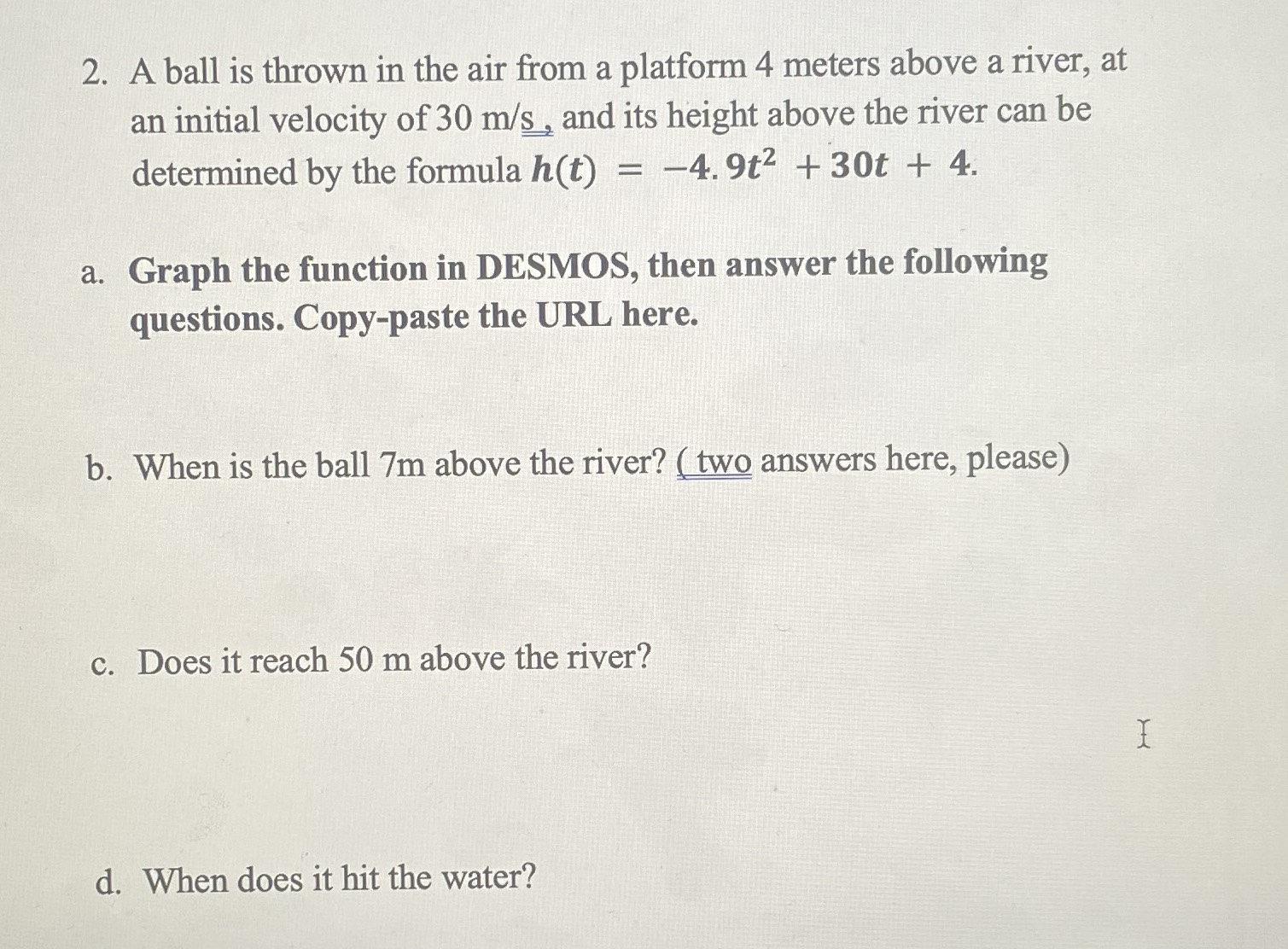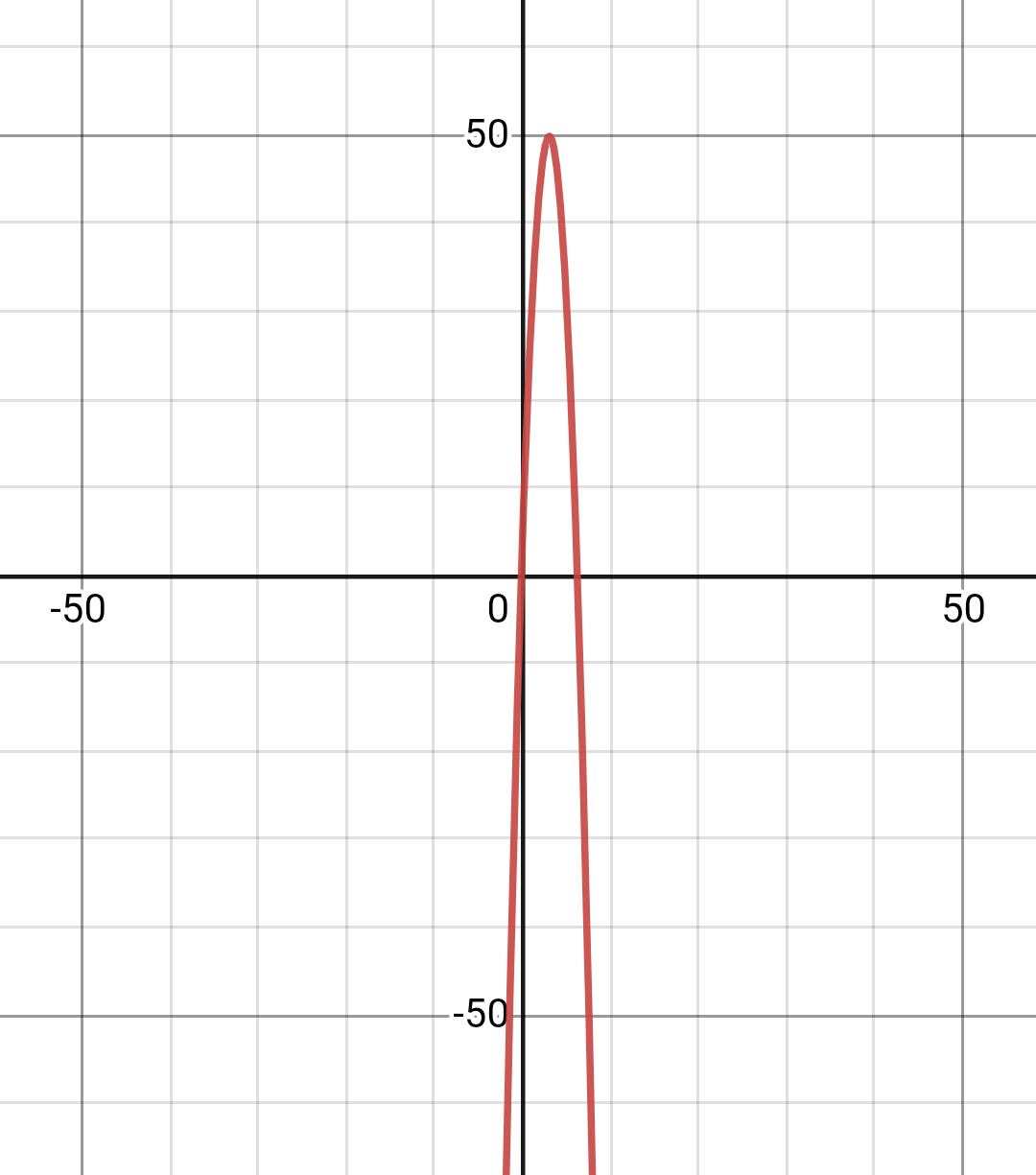### ¿Todavía tienes preguntas de matemáticas?

Pregunte a nuestros tutores expertos
Algebra
PreguntaA ball is thrown in the air from a platform $$4$$ meters above a river, at an initial velocity of $$30 m / s$$ , and its height above the river can be determined by the formula $$h ( t ) = - 4.9 t ^ { 2 } + 30 t + 4$$ .

a. Graph the function in DESMOS, then answer the following questions. Copy-paste the URL here.

b. When is the ball $$7 m$$ above the river? (two answers here, please)

c. Does it reach $$50 m$$ above the river?

d. When does it hit the water?

a.